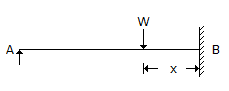# Civil Engineering - UPSC Civil Service Exam Questions - Discussion

45.

Consider the following statements :
In the beam shown in the given figure, for all positions of load W (except x = 0)
1. bending moment is maximum at B
2. bending moment is maximum under load
3. deflection is zero at A
4. deflection is zero at B
Of these statements :[A]. 1 and 3 are correct [B]. 2 and 4 are correct [C]. 1 and 4 are correct [D]. 1, 3 and 4 are correct

Explanation:

No answer description available for this question.

 Phani said: (Mar 8, 2018) Option C is correct I think.

 Nouman said: (Apr 8, 2020) Option "C" could not be considered as correct because this is not a cantilever. As there is a vertical reaction at support "A", so option "D" is correct.